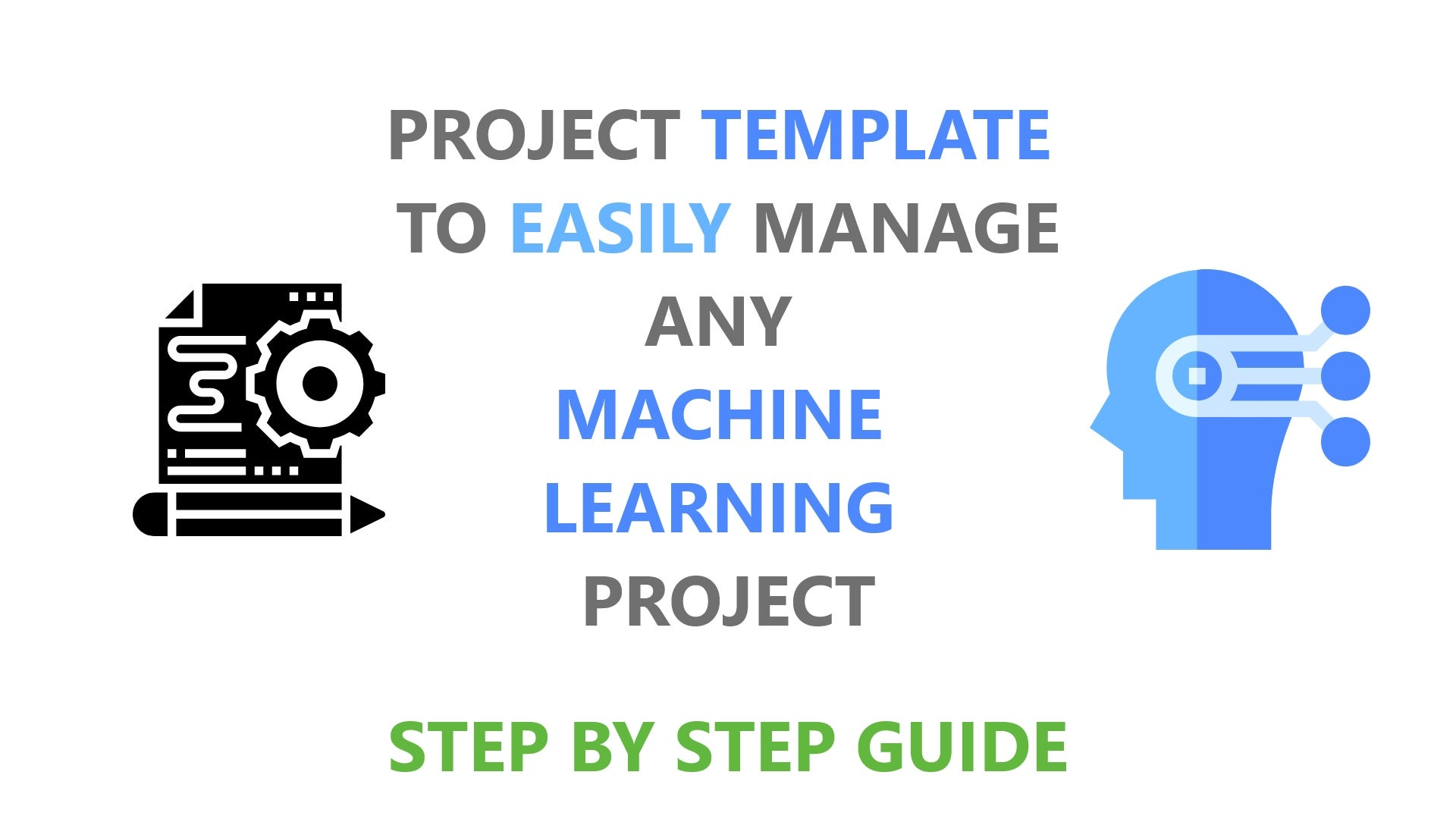# Machine learning project template : The right way..

Original article was published by Yug Damor on Deep Learning on Medium# Machine learning project template : The right way..

IN THIS POST I AM GOING TO PROVIDE STEP BY STEP GUIDE TO HANDLE ANY MACHINE LEARNING PROJECT.

SO I WILL LIST DOWN EACH AND EVERY STEP WHICH YOU HAVE TO FOLLOW IN ANY ML PROJECT.

## STEP 1 : Prepare Problem OR Understand the problem statement

In this step you have to understand your problem statement.

in this step you also have to understand the dataset.you have to observe your dataset.

example : you need to import pandas in order to load the dataset

here you need to load the dataset using pandas.

## STEP 2 : Summarize Data

here you have to check the Descriptive statistics :

• the distribution
• the central tendency
• the dispersion

you have to observe the distribution of data . then cnetral tendency.

and in this step you can also do data visualizations if required.

## STEP 3 : Prepare Data

A. Data Cleaning

in this step you have to remove or modify data that is incorrect, incomplete, irrelevant, duplicated, or improperly formatted.

B. Feature Selection

select those features which contribute most to your prediction variable or output in which you are interested in.

Having irrelevant features in your data can decrease the accuracy of the models and make your model learn based on irrelevant features.

C. Data Transforms

• Converting non-numeric features into numeric. You can’t do matrix multiplication on a string, so we must convert the string to some numeric representation.
• Resizing inputs to a fixed size. Linear models and feed-forward neural networks have a fixed number of input nodes, so your input data must always have the same size. For example, image models need to reshape the images in their dataset to a fixed size.
• Tokenization or lower-casing of text features.
• Normalized numeric features (most models perform better afterwards).
• Allowing linear models to introduce non-linearities into the feature space.

D. Split your data into train and test set

## STEP 4 : Evaluate Algorithms

Different machine learning algorithms search for different trends and patterns. One algorithm isn’t the best across all data sets or for all use cases. To find the best solution, you need to conduct many experiments, evaluate machine learning algorithms, and tune their hyperparameters.

in this step you have to train multiple ml models.

A. Train Your model on training dataset

B. evaluation of model

• Evaluating a model is a core part of building an effective machine learning model
• There are several evaluation metrics, like confusion matrix, cross-validation, AUC-ROC curve, etc.
• Different evaluation metrics are used for different kinds of problems.

C. Spot Check Algorithms

this is technique in applied machine learning designed to quickly and objectively provide a first set of results on a new predictive modeling problem.

• Spot-checking provides a way to quickly discover the types of algorithms that perform well on your predictive modeling problem.
• How to develop a generic framework for loading data, defining models, evaluating models, and summarizing results.
• How to apply the framework for classification and regression problems.

D. Compare Algorithms

1. Space complexity
3. Online and Offline
4. etc
• Online and offline learning refers to the way a machine learning software learns to update the model

## STEP 5 : Improve Accuracy

A. Algorithm Tuning

We know that machine learning algorithms are driven by parameters. These parameters majorly influence the outcome of learning process.

The objective of parameter tuning is to find the optimum value for each parameter to improve the accuracy of the model. To tune these parameters, you must have a good understanding of these meaning and their individual impact on model. You can repeat this process with a number of well performing models.

B. Ensembles

This is the most common approach found majorly in winning solutions of Data science competitions. This technique simply combines the result of multiple weak models and produce better results. This can be achieved through many ways:

• Bagging (Bootstrap Aggregating)
• Boosting

## STEP : 6 Finalize Model

A. Create standalone model on entire training dataset

B. Save model for later use

thank you.